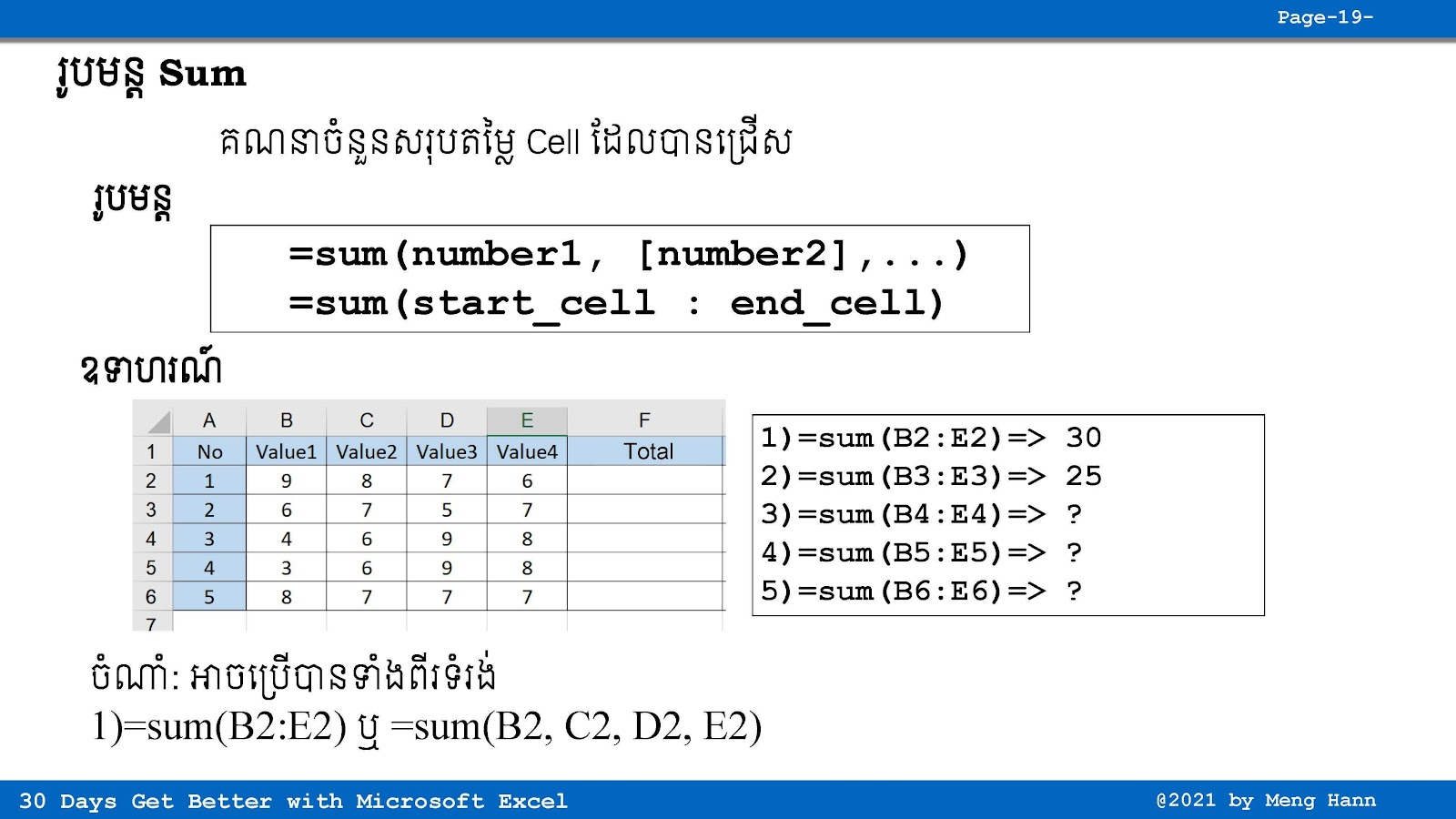# 13. How to use SUM in Excel

13. How to use SUM in Excel speak Khmer
This Excel tutorial in Khmer show how to use SUM formula step by step. SUM is calculate total values in Excel.
SUM Formula
=SUM(Number1,[Number2],...)
=SUM(start_cell:end_cell)
◾ Number1: Required, value to calculate, (number1,...,number255)
◾ start_cell: Required, start value to calculate.
◾ end_cell: Required, end value to calculate.